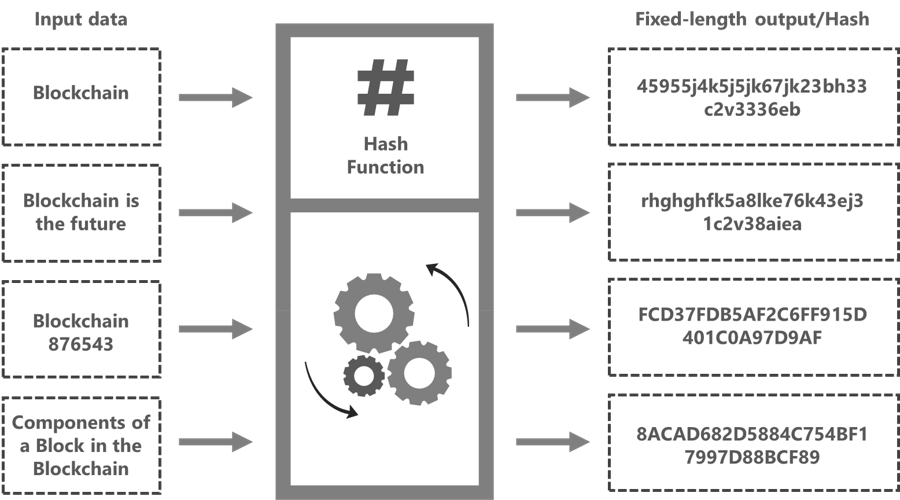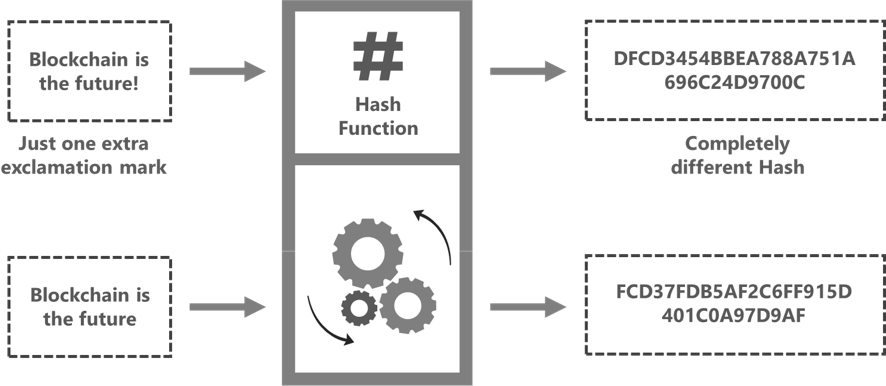# Hash Functions in Blockchain (Part 3- Blockchain Series)

Welcome to the third part of the 100 part series on Blockchain.

Part 1: What is Blockchain technology?

Part 2: Components of a Block in the Blockchain

Hash functions are one of the most extensively-used cryptographic algorithms that generate a fixed-length output for any input data irrespective of its size and length. The input data can be a word, a sentence, a longer text, or an entire file. The fixed-length output generated for the input data is called a hash. Many types of cryptographic hash functions/ algorithms are available like MD5, BLAKE2, SHA-1, SHA-256, etc. Secure Hashing Algorithm 256, commonly referred to as SHA-256, is one of the most famous cryptographic hash functions used extensively in Blockchain technology. It was developed by the National Security Agency (NSA) in 2001.Hash or fixed-length output for any input data

When we pass a certain message through the hash algorithm, it generates a hash against this input. Regardless of the size of the letters or numbers you input, the hash algorithm always generates a fixed-length output. The fixed-length output can vary like 32-bit, 64-bit, 128-bit, or 256-bit depending on the hash algorithm being used. For instance, SHA-256 generates a hash value of 256 bits, equivalent to the size of 64 characters.

Using a fixed-length output increases security since anyone trying to decrypt the hash won’t be able to tell how long or short the input is simply by looking at the length of the output. The only method to determine the original string from its hash is by using “brute-force.” Brute-force basically means that one has to take random inputs, hash them and compare them with the target hash. For instance, if the SHA-256 hash algorithm is used, a brute-force attack would need to make 2^256 attempts to generate the initial data.

Now, let’s discuss the important properties of a cryptographic hash function used in the Blockchain:

# A small change in the input value alters the Hash value significantly

One of the unique properties of a cryptographic hash function is that even a small change in the input value brings about a drastic change in its output value. This is referred to as the Avalanche Effect. For instance, when the first input, ‘Blockchain is the future’ is passed through the hash function, a specific output or hash is generated. But when a small change is made to the input, like, an extra exclamation mark is added- ‘Blockchain is the future!’ you can see that the new hash is generated, which is entirely different from the previous hash. This property of cryptographic hash functions makes them resistant to hacking as no correlation can be derived about the input data by just looking at the hash alone.Different hash is generated when a small change is made in input data

# Computationally Efficient

One of the other crucial properties of a cryptographic hash function used in the Blockchain is its quick computation. The hash function requires computers on the Blockchain network to perform certain complex mathematical tasks to generate a hash from the input data. So when we say that the hash function should be computationally efficient, it simply means that the computers should be able to finish the required mathematical task in a short time.

# Deterministic

A cryptographic hash function used in the Blockchain must be deterministic. In simple words, a hash function is said to be deterministic if it generates the same hash whenever the same input is passed through it. No matter how many times we pass an input ‘ Blockchain is the future’ through the hash function, it should always generate the same exact output or hash every single time.

If different outputs are generated by a hash function for the same input, the hash function will become useless, and it would be impossible to verify a specific input.

# Pre-Image Resistance

The input for a cryptographic hash function can be any kind of data. This data can be a number, a word, a sentence, a passcode, a song, a book, or a complete movie. But the hash generated for any kind of input data by the hashing algorithm will be an alphanumeric code and that too of a fixed length.

It is very crucial for a cryptographic hash function to be Pre-image resistant. Pre-image resistance means that the output generated by a cryptographic hash function must not reveal any information about the input data. For example, when an input X is passed through the hash function, the hash generated is represented as H(X). Pre-image resistance simply means that even if you know H(X), it must be infeasible for you to determine the corresponding input X.

# Collision Resistant

Collision resistance is another important property of a cryptographic hash function. Being collision-resistant simply means that it should be highly improbable to generate the same output or hash for two different inputs.

As discussed earlier, the input for a hash function can be of any type, size, and length. Therefore, there are infinite possibilities for the data input that can be fed into a hash function. But the corresponding hash or output generated will have a fixed length. This means that there will be a finite number of outputs that can be generated using the hash algorithm. If inputs can be infinite and outputs are finite in number, it is quite possible that more than one input can produce the same output.

So the goal of being collision-resistant is to make the probability of finding any two such inputs which share the same output negligible. So if a hashing function is collision-resistant, this possibility won’t pose any security risk to the data.

# One-way functions

Hash functions are generally referred to as one-way functions because they are not reversible. While a hash function is a cryptographic function, it’s not encryption. Encryption works by encrypting the relevant data with an encryption algorithm and an encryption key. This results in a ciphertext that can only be viewed in its original form if decrypted with the correct key. A hash function, in contrast to encryption, works as a one-way function; in simple words, if you have a hash, you can not decrypt it to find the corresponding input. So in a real-life scenario, even if a hacker gets access to a hash output, it is completely useless as he can’t decrypt it to get the input.